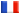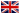# Séminaire de Cryptographie

## Alan Lauder### Deformation theory and the computation of zeta functions

The problem of computing the zeta function of a variety over a finite field has attracted considerable interest in recent years, motivated in part by an application in cryptography. (In less fancy language, the problem is just to compute the number of solutions to a system of polynomial equations over a finite field.) I will discuss a new algorithm for computing zeta functions which is based upon relative p-adic cohomology. The idea is that to compute the zeta function of a single projective hypersurface, say , one puts it in a one-dimensional family of hypersurfaces. As one moves through this family, the zeta function varies in a manner which is controlled by a differential equation. One can arrange matters so that one fibre in the family has an easily computed zeta function. By solving the differential equation locally around this fibre, and using a form of analytic continuation, one can now recover the zeta function of any fibre in the family. In particular, one gets the zeta function of the original hypersurface! The key point is that because the `deformation' from the original hypersurface to the easy one is one-dimensional, the complexity of this approach is largely independent of the dimension of the hypersurface. In fact, one gets a uniform dependence on the input size over all dimensions. This contrasts starkly with existing approaches, whose performance deteriorates as the dimension increases. I believe the talk should be of interest to both cryptographers and p-adic cohomologists.